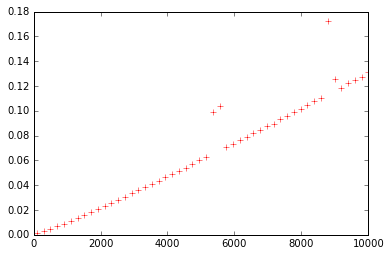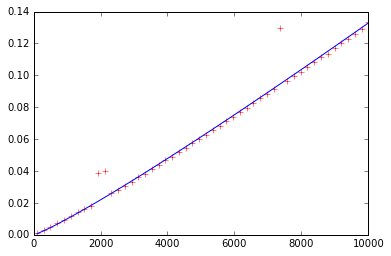# Priority Queues¶

## Agenda¶

1. Motives
2. Naive implementation
3. Heaps
• Mechanics
• Implementation
• Run-time Analysis
4. Heapsort

## 1. Motives¶

Prior to stacks & queues, the sequential data structures we implemented imposed an observable total ordering on all its elements, which were also individually accessible (e.g., by index).

Stacks & Queues restrict access to elements (to only 1 insertion/deletion point), thereby simplifying their implementation. They don't, however, alter the order of the inserted elements.

Data structures that impose a total ordering are useful — e.g., one that maintains all elements in sorted order at all times might come in handy — but their design and implementation are necessarily somewhat complicated. We'll get to them, but before that ...

Is there a middle ground? I.e., is there a place for a data structure that restricts access to its elements, yet maintains an implied (though not necessary total) ordering?

### "Priority Queue"¶

Like a queue, a priority queue has a restricted API, but each element has an implicit "priority", such that the element with the highest ("max") priority is always dequeued, regardless of the order in which it was enqueued.

## 2. Naive implementation¶

In :
class PriorityQueue:
def __init__(self, key=lambda x:x):
self.data = []
self.key = key

self.data.append(x)
self.data.sort(key=self.key)

def max(self):
return self.data[-1]

def pop_max(self):
return self.data.pop()

def __bool__(self):
return len(self.data) > 0

def __len__(self):
return len(self.data)

def __repr__(self):
return repr(self.data)

In :
pq = PriorityQueue()

In :
import random
for _ in range(10):

In :
pq

Out:
[1, 2, 42, 68, 69, 71, 74, 78, 85, 95]
In :
while pq:
print(pq.pop_max())

95
85
78
74
71
69
68
42
2
1

In :
pq = PriorityQueue(key=lambda x:-x)
for _ in range(10):
pq

Out:
[74, 60, 58, 44, 39, 34, 23, 23, 15, 5]
In :
pq = PriorityQueue(key=lambda s:len(s))
pq

Out:
['hi', 'hola', 'hello']

## 3. Heaps¶

### Mechanics¶

In an ordered, linear structure, inserting an element changes the positions of all of its successors, which include all elements at higher indices (positions).

Reframing the problem: how can we reduce the number of successors of elements as we move through them? (Consider analogy: we don't think of all the organisms in the world as belonging to one gigantic, linear list! How do we reduce the number we have to consider when thinking about certain characteristics?)

Use a hierarchical structure! A tree.

### Implementation¶

In :
class Heap:
def __init__(self):
self.data = []

@staticmethod
def _parent(idx):
return (idx-1)//2

@staticmethod
def _left(idx):
return idx*2+1

@staticmethod
def _right(idx):
return idx*2+2

def _heapify(self, idx=0):
while True:
l = Heap._left(idx)
r = Heap._right(idx)
maxidx = idx
if l < len(self) and self.data[l] > self.data[idx]:
maxidx = l
if r < len(self) and self.data[r] > self.data[maxidx]:
maxidx = r
if maxidx != idx:
self.data[idx], self.data[maxidx] = self.data[maxidx], self.data[idx]
idx = maxidx
else:
break

def _heapify_rec(self, idx=0):
l = Heap._left(idx)
r = Heap._right(idx)
maxidx = idx
if l < len(self) and self.data[l] > self.data[idx]:
maxidx = l
if r < len(self) and self.data[r] > self.data[maxidx]:
maxidx = r
if maxidx != idx:
self.data[idx], self.data[maxidx] = self.data[maxidx], self.data[idx]
self._heapify(maxidx)

self.data.append(x)
i = len(self.data) - 1
p = Heap._parent(i)
while i > 0 and self.data[p] < self.data[i]:
self.data[p], self.data[i] = self.data[i], self.data[p]
i = p
p = Heap._parent(i)

def max(self):
return self.data

def pop_max(self):
ret = self.data
self.data = self.data[len(self.data)-1]
del self.data[len(self.data)-1]
self._heapify()
return ret

def __bool__(self):
return len(self.data) > 0

def __len__(self):
return len(self.data)

def __repr__(self):
return repr(self.data)

In :
h = Heap()

In :
import random
for _ in range(15):

In :
h

Out:
[98, 98, 90, 15, 86, 67, 41, 0, 13, 36, 41, 26, 30, 32, 4]
In :
while h:
print(h.pop_max())

98
98
90
86
67
41
41
36
32
30
26
15
13
4
0


## 4. Heapsort¶

In :
def heapsort(iterable):
heap = Heap()
for x in iterable:
sorted_lst = []
while heap:
sorted_lst.append(heap.pop_max())
sorted_lst.reverse()
return sorted_lst

In :
import random

def pairs(iterable):
it = iter(iterable)
a = next(it)
while True:
b = next(it)
yield a,b
a = b

lst = heapsort(random.random() for _ in range(1000))
all((a <= b) for a, b in pairs(lst))

Out:
True
In :
import timeit
def time_heapsort(n):
return timeit.timeit('heapsort(rlst)',
'from __main__ import heapsort; '
'import random; '
'rlst = (random.random() for _ in range({}))'.format(n),
number=1000)

In :
%matplotlib inline
import matplotlib.pyplot as plt
import numpy as np

ns = np.linspace(100, 10000, 50, dtype=np.int_)
plt.plot(ns, [time_heapsort(n) for n in ns], 'r+')
plt.show()In :
%matplotlib inline
import matplotlib.pyplot as plt
import numpy as np

ns = np.linspace(100, 10000, 50, dtype=np.int_)
plt.plot(ns, [time_heapsort(n) for n in ns], 'r+')
plt.plot(ns, ns*np.log2(ns)*0.01/10000, 'b') # O(n log n) plot
plt.show()